### 5.4Complex Logic Functions Using Improved Symmetric Implication

In the symmetricized MRAM-based implication logic architecture (Fig. 5.6a), complex logic functions are implemented by using subsequent FALSE (TRUE) and IMP (NIMP) operations. Regardless of the number of inputs, only two extra (work) memory elements  are needed to compute all Boolean logic functions with maximum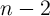inputs in an array with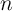1T/1MTJ cells. Nevertheless, in different logic function designs presented in the following we try to minimize the error probabilities and the total logic steps (equivalent to the total operation time and energy consumption) rather than the number of required extra memory elements (equivalent to area), since two cells out of kilobytes or megabytes are negligible.

As an example, in the implication logic the XOR function can be designed as :

 a1 ⊕ a2 ≡ a1.a2 + a1.a2 ≡ (a1 IMP a2) IMP (a2 NIMP a1). (5.6)

In the MRAM logic architecture, its implementation (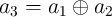) comprises the following sequential steps (Eq. 5.7) on four MTJs, where two MTJs are inputs (and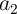) and two MTJs act as work MTJs.

 TRUE : a3 = 1 NIMP : a3 → a1 ≡{a3′ = a 3.a1 = a1} TRUE : a4 = 1 NIMP : a4 → a2 ≡{a4′ = a 4.a2 = a2} NIMP : a2 → a1 ≡{a2′ = a 2.a1} NIMP : a4 → a3 ≡{a4′ = a 4.a3 = a2.a1} TRUE : a1 = 1 NIMP : a1 → a2 ≡{a1′ = a 1.a2 = 1.(a2.a1) = a2 + a1} NIMP : a1 → a4 ≡{a1′ = a 1.a4 = (a2 + a1).(a2 + a1)} TRUE : a3 = 1 NIMP : a3 → a1 ≡{a3′ = a 3.a1 = a2.a1 + a2.a1 ≡ a1 ⊕ a2} (5.7)

According to Eq. 4.27, as the implementation includes seven NIMP operations, the reliability of the implication-based XOR for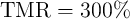is obtained as

 EXOR = 1 - (1 -EIMP)7 ≃ 6.5 × 10-4. (5.8)
This is about one order of magnitude smaller than that of the most reliable design with the reprogrammable architecture for the same MTJ device characteristics and it requires the same number of sequential steps compared to its reprogrammable counterpart comprising 11 operations (Eq. 5.4).

#### 5.4.1Non-Volatile Logic Fan-Out

In the magnetoresistive (MR) non-volatile logic the resistance states of the MR devices are the physical state variables. This is different compared to CMOS logic where information is represented by charge or voltage. Most of the previous proposals for MR-based logic circuits [195, 196, 19, 197, 51, 198, 53, 192] require intermediate circuitry for sensing the data stored in each non-volatile magnetic element to implement fan-out functions . This increases the power consumption, time delay, area, and integration complexity. A possible remedy is to switch to direct communication between the MR devices thus removing intermediate circuitry [55, 199, 200, 26, 56, 201]. However, this makes the computations localized by confining them to the MR devices which are directly coupled. Therefore, in the state-of-the-art, large-scale integration of complex logic functions is difficult or may be even impossible by using the non-volatile logic-in-memory concept due to the hard linking between different gates and the need for sensing amplifiers and intermediate circuitry. In the STT-MRAM-based implication logic with symmetric implementation (Fig. 5.6a), the issue of the non-volatile logic fan-out function can be addressed as follows.

The output information of a logic operation (IMP/NIMP) can be used to perform the next operation with an arbitrary MTJ in the array as a source or a target input. This provides high flexibility with regard to the non-volatile logic fan-out function. However, when the (N)IMP operation is executed, the target data is not available anymore, as the (N)IMP result is written into the target MTJ. Therefore, as long as the data is used only as the source data in the subsequent operations, multiple logic fan-output is not required. But, when the data has to be reused after being the target data of an operation, implication-based NOT and COPY (2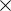NOT) operations are executed to keep the data available. As a consequence, when multiple fan-out is required, a set of FALSE (TRUE) and IMP (NIMP) operations are performed to execute NOT and COPY operations in the implication MRAM array (Fig. 5.6a). This allows to copy information from the source MTJ (which could be the target MTJ of the previous operation) to an arbitrary target MTJ in the array without the need for intermediate sensing. As an example, the next section describes the STT-MRAM-based implementation in the implication logic framework.

#### 5.4.2Stateful STT-MRAM-based Full Adder

A full adder is a basic element of arithmetic circuits. As is well known, it adds three binary inputs (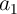,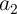, and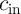) and produces two binary outputs, sum (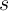) and carry () obtained as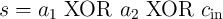(5.9)

and(5.10)

Since the implication gates cannot fan-out, a logical value which is required as the target variable for an implication operation has to be copied in a work (additional) cell (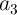,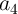, or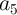), if it is needed as an input for subsequent operations. Eq. 5.12 and Eq. 5.13 show the TRUE/NIMP and the FALSE/IMP-based implementations of a stateful full adder using the MRAM implication logic arrays, respectively. The detailed derivations are given in Appendix A.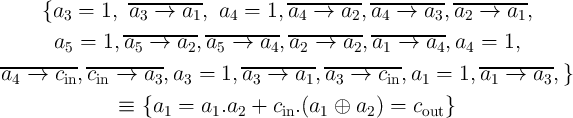(5.11)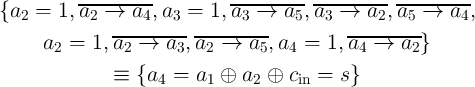(5.12)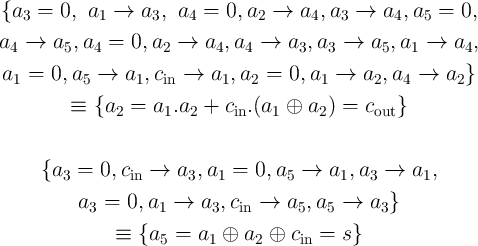(5.13)

The FALSE/IMP-based design involves only 27 subsequent (9 FALSE and 18 IMP) operations on three input cells (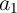,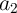, and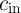) and three additional cells (,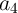, or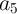), in contrast to the earlier proposed implication-based scheme  with 19 FALSE and 18 IMP operations (37 total) for generating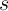and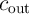, respectively, and four additional cells. Therefore, this design decreases the total implementation time by about 30% and reduces energy consumption and device count (area). As ‘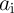NIMP’ and ‘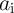NIMP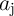’ are equivalent to ‘NOT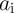and ‘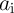AND (NOT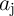)’, respectively, some operations can be omitted to minimize the total effort.

In the magnetic full adders based on the logic-in-memory architecture presented in  and , the MTJs are used only as ancillary devices which store the result of the logic computations performed by the transistors. Therefore, the logic operations are still performed by CMOS logic elements and 26 transistors for logic, 8 for MTJ writing, and 4 MTJs for storing data are required. In contrast, the MRAM-based implication architecture exploits the MTJs as the main devices for computations and eliminates the need for extra logic gates. It therefore brings considerable benefit regarding the device count. Furthermore, a key limitation of the magnetic full adders in  and  is the necessity of different kind of inputs and outputs for which some inputs or outputs are voltage signals, whereas the others are the resistance state of the MTJ elements. This mismatch causes the need for extra hardware and increases complexity.